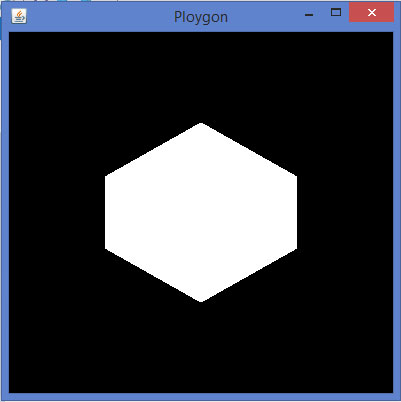# JOGL - Pre Defined Shapes

In the Previous chapters we have learned how draw a shapes such as line, triangle, rhombus using JOGL. We draw lines by passing a predefined field, Gl_lines to glBegin() method.

Other than GL_LINES, the glBegin() method accepts eight more parameters. You can use them to draw different shapes. These are used the same way as GL_LINES.

The following table shows the glBegin() method parameters along with their description −

Sr.No Parameters and Description
1

GL_LINES

Creates each pair of vertices as an independent line segment.

2

GL_LINE_STRIP

Draws a connected group of line segments from the first vertex to the last.

3

GL_LINE_LOOP

Draws a connected group of line segments from the first vertex to the last, again back to the first.

4

GL_TRIANGLES

Treats each triplet of vertices as an independent triangle.

5

GL_TRIANGLE_STRIP

Draws a connected group of triangles. One triangle is defined for each vertex presented after the first two vertices.

6

GL_TRIANGLE_FAN

Draws a connected group of triangles. One triangle is defined for each vertex presented after the first two vertices.

7

Treats each group of four vertices as an independent quadrilateral.

8

Draws a connected group of quadrilaterals. One quadrilateral is defined for each pair of vertices presented after the first pair.

9

GL_POLYGON

Draws a single, convex polygon. Vertices 1,…,n define this polygon.

Let us see some examples using glBegin() parameters.

### Program to draw a Line Strip

```import javax.media.opengl.GL2;
import javax.media.opengl.GLAutoDrawable;
import javax.media.opengl.GLCapabilities;
import javax.media.opengl.GLEventListener;
import javax.media.opengl.GLProfile;
import javax.media.opengl.awt.GLCanvas;

import javax.swing.JFrame;

public class LineStrip implements GLEventListener {

@Override
public void display(GLAutoDrawable drawable) {

final GL2 gl = drawable.getGL().getGL2();

gl.glBegin (GL2.GL_LINE_STRIP);
gl.glVertex3f(-0.50f,-0.75f, 0);
gl.glVertex3f(0.7f,0.5f, 0);
gl.glVertex3f(0.70f,-0.70f, 0);
gl.glVertex3f(0f,0.5f, 0);
gl.glEnd();
}

@Override
public void dispose(GLAutoDrawable arg0) {
//method body
}

@Override
public void init(GLAutoDrawable arg0) {
// method body
}

@Override
public void reshape(GLAutoDrawable arg0, int arg1, int arg2, int arg3, int arg4) {
// method body
}

public static void main(String[] args) {

//getting the capabilities object of GL2 profile
final GLProfile profile = GLProfile.get(GLProfile.GL2);
GLCapabilities capabilities = new GLCapabilities(profile);

// The canvas
final GLCanvas glcanvas = new GLCanvas(capabilities);
LineStrip r = new LineStrip();
glcanvas.setSize(400, 400);

//creating frame
final JFrame frame = new JFrame ("LineStrip");

frame.setSize(frame.getContentPane().getPreferredSize());
frame.setVisible(true);

}//end of main

}//end of classimport javax.media.opengl.GL2;
```

If you compile and execute the above code, the following output is generated −### Code snippet for display() method to draw a Line Loop

```public void display(GLAutoDrawable drawable) {

final GL2 gl = drawable.getGL().getGL2();

gl.glBegin (GL2.GL_LINE_LOOP);

gl.glVertex3f( -0.50f, -0.75f, 0);
gl.glVertex3f(0.7f, .5f, 0);
gl.glVertex3f(0.70f, -0.70f, 0);
gl.glVertex3f(0f, 0.5f, 0);

gl.glEnd();
}
```

If you replace the display() method of any of the basic template programs with the above code, compile, and execute it, the following output is generated −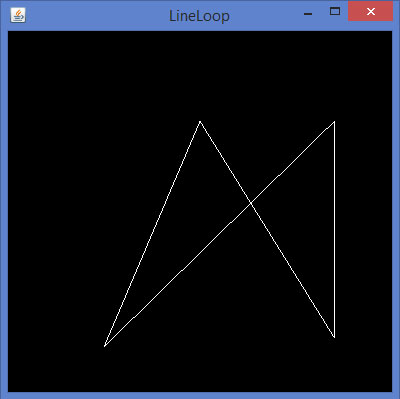### Code snippet for display() method to draw a triangle using GL_TRIANGLES

```public void display(GLAutoDrawable drawable) {

final GL2 gl = drawable.getGL().getGL2();

gl.glBegin(GL2.GL_TRIANGLES);        // Drawing Using Triangles

gl.glVertex3f(0.5f,0.7f,0.0f);       // Top
gl.glVertex3f(-0.2f,-0.50f,0.0f);    // Bottom Left
gl.glVertex3f(0.5f,-0.5f,0.0f);      // Bottom Right

gl.glEnd();
}
```

If you replace the display() method of any of the basic template programs with the above code, compile, and execute it, the following output is generated −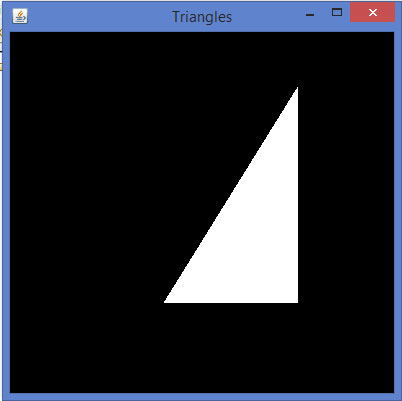### Code snippet for display() method to draw a Triangle Strip

```public void display(GLAutoDrawable drawable) {

final GL2 gl = drawable.getGL().getGL2();

gl.glBegin (GL2.GL_TRIANGLE_STRIP);

gl.glVertex3f(0f,0.5f,0);
gl.glVertex3f(-0.50f,-0.75f,0);
gl.glVertex3f(0.28f,0.06f,0);
gl.glVertex3f(0.7f,0.5f,0);
gl.glVertex3f(0.7f,-0.7f,0);

gl.glEnd();
}
```

If you replace the display() method of any of the basic template programs with the above code, compile and execute it, the following output is generated −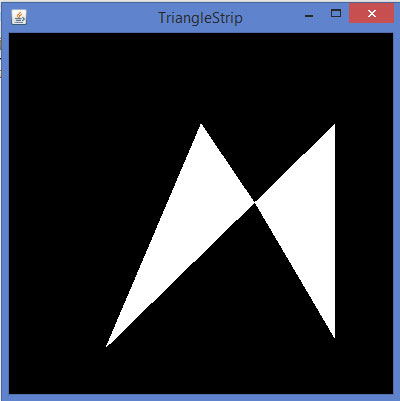### Code snippet for display() method to draw a quadrilateral

```public void display(GLAutoDrawable drawable) {

final GL2 gl = drawable.getGL().getGL2();

gl.glVertex3f( 0.0f,0.75f,0);
gl.glVertex3f(-0.75f,0f,0);
gl.glVertex3f(0f,-0.75f,0);
gl.glVertex3f(0.75f,0f,0);

gl.glEnd();
}
```

If you replace the display() method of any of the basic template programs with the above code, compile, and execute it, the following output is generated −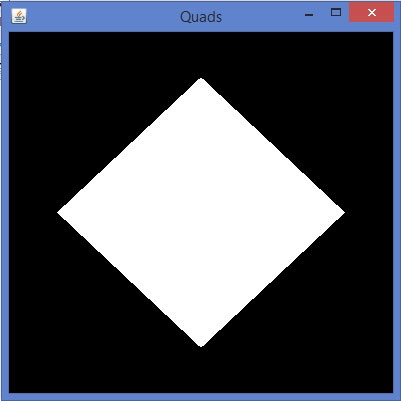### Code snippet for display() method to draw a polygon

```public void display(GLAutoDrawable drawable) {

final GL2 gl = drawable.getGL().getGL2();

gl.glBegin(GL2.GL_POLYGON);

gl.glVertex3f(0f,0.5f,0f);
gl.glVertex3f(-0.5f,0.2f,0f);
gl.glVertex3f(-0.5f,-0.2f,0f);
gl.glVertex3f(0f,-0.5f,0f);
gl.glVertex3f(0f,0.5f,0f);
gl.glVertex3f(0.5f,0.2f,0f);
gl.glVertex3f(0.5f,-0.2f,0f);
gl.glVertex3f(0f,-0.5f,0f);

gl.glEnd();
}
```

If you replace display() method of any of the basic template programs with the above code, compile, and execute it, the following output is generated −